Next: Buoyancy Up: Hydrostatics Previous: Introduction

# Hydrostatic Pressure

Consider a body of water which is stationary in a reference frame that is fixed with respect to the Earth's surface. In this chapter, such a frame is treated as approximately inertial. Letmeasure vertical height, and suppose that the region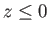is occupied by water, and the region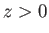by air. According to Equation (1.79), the air/water system remains in mechanical equilibrium (i.e.,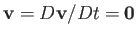) provided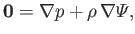(2.1)

whereis the static fluid pressure,the mass density,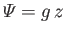the gravitational potential energy per unit mass, and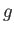the (approximately uniform) acceleration due to gravity. Now,(2.2)

where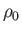is the (approximately uniform) mass density of water. Here, the comparatively small mass density of air has been neglected. Because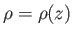and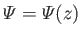, it immediately follows, from Equation (2.1), that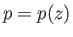, where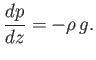(2.3)

We conclude that constant pressure surfaces in a stationary body of water take the form of horizontal planes. Making use of Equation (2.2), the previous equation can be integrated to give(2.4)

where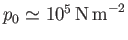is atmospheric pressure at ground level (Batchelor 2000). According to this expression, pressure in stationary water increases linearly with increasing depth (i.e., with decreasing, for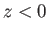). In fact, given that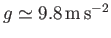and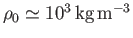(Batchelor 2000), we deduce that hydrostatic pressure in water rises at the rate of 1 atmosphere (i.e.,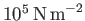) every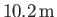increase in depth below the surface.Next: Buoyancy Up: Hydrostatics Previous: Introduction
Richard Fitzpatrick 2016-03-31